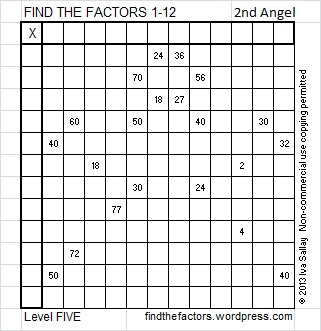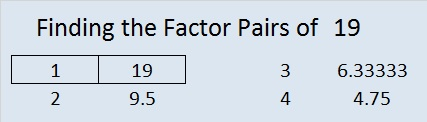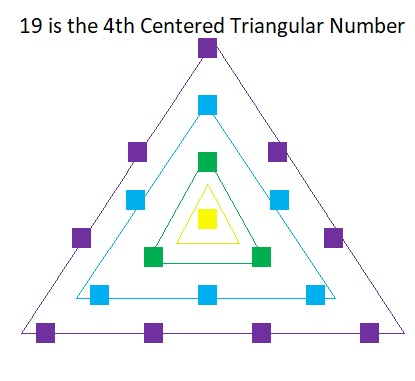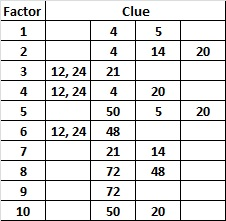### Today’s Puzzle and a Last-minute Gift Idea:

It’s Christmas Eve or even Christmas day, and maybe all of your shopping didn’t get done. Maybe you didn’t want to drive anyplace because of bad weather, or your favorite stores were closed early for the holiday. Well, if someone on your list likes number-placing puzzles (like Sudoku or Kakuro), then I have a last-minute gift idea for you, and it’s free. I design a number-placing logic puzzle based on the multiplication table called FIND THE FACTORS. If you have a computer, the internet, and a printer, you can print a little holiday booklet filled with these puzzles and give it as a gift. If the person on your gift list is many miles away, you can even send the booklet electronically. This last-minute gift is good for the brain and can be good for the memory. The level 1 and level 2 puzzles can be solved by children 3rd grade and up, but most of the higher level puzzles will be challenging for everyone regardless of age.

Here is a  puzzle created to look a little like an angel just for the holidays:To solve the puzzle above simply write the numbers 1 – 12 in the top row and also in the first column so that those numbers are the factors of the given clues. Okay, maybe it isn’t quite that simple. You have to know basic multiplication facts and use logic to figure out where the numbers go, and yes, I may try to trick you. But you and the people on your gift list have enough skills and persistence to find the one and only correct solution.

Now glancing at the puzzle above you may think you know all the answers, but…

This is what the solved puzzle looks like. Some of those factors may surprise you. That is why using logic is so important when solving the puzzles. (Once the factors are found, filling out the rest of the table is optional.)Click 2013 Factor Holiday to download a copy of the puzzle booklet. Some of the puzzles in the booklet are a little easier than the one above because they are a lower level or they only use factors up to 10. Have a very Merry Christmas and a Happy New Year!

### Factors of the Number 19:

• 19 is a prime number.
• Prime factorization: 19 is prime.
• The exponent of prime number 19 is 1. Adding 1 to that exponent we get (1 + 1) = 2. Therefore 19 has exactly 2 factors.
• Factors of 19: 1, 19
• Factor pairs: 19 = 1 x 19
• 19 has no square factors that allow its square root to be simplified. √19 ≈ 4.3588989How do we know that 19 is a prime number? If 19 were not a prime number, then it would be divisible by at least one prime number less than or equal to √19 ≈ 4.4. Since 19 cannot be divided evenly by 2 or 3, we know that 19 is a prime number.

19 is never a clue in the FIND THE FACTORS puzzles.

### More About the Number 19:

19 is the fourth centered triangular number. There are 19 squares in the graphic below:Why? Because 1 + 3(1) + 3(2) + 3(3) = 19.

19 is also the third centered hexagonal number.
Why? Because 1 + 6(1) + 6(2) = 19.
Imagine lines forming concentric hexagons in the drawing from the tweet below:

Related articles with other ideas for last-minute gifts:

# 10 Just Like Sudoku?

10 is a composite number. 10 = 1 x 10 or 2 x 5. Factors of 10: 1, 2, 5, 10. Prime factorization: 10 = 2 x 5.When 10 is a clue in the FIND THE FACTORS puzzles, either 1 x 10 or else 2 x 5 will work for that particular puzzle.

On numerous occasions when I have demonstrated how to solve a Find the Factors puzzle, someone will remark that the puzzle is just like Sudoku. What are common factors that both a Find the Factors puzzle and a Sudoku puzzle will have? 1) Both will have only one solution. 2) Both require the solver to be able to count, write, and place the numerals 1 to 9, but Find the Factors also requires the number 10 be placed. 3) Both were originally designed to require logic to be solved. 4) Both puzzles utilize a square grid. 5) Both puzzles have several difficulty levels and variations that make the puzzles more challenging.

What factors do the two puzzles NOT have in common? 1) A difficult Sudoku puzzle can take some people almost an hour to solve while Find the Factors  would never take that long. 2) Sudoku has been a wildly popular puzzle while Find the Factors is known only among a small circle of people who have had some kind of contact with me. 3) Some more recent Sudoku puzzles require the solvers to guess and check which is getting away from its logic puzzle roots and is making it less popular for some people.  4) Sudoku could just as easily be made with letters of an alphabet, colors, or the names of the planets (if you include Pluto), while Find the Factors has to be made with numbers. 5) Sudoku requires only counting, while Find the Factors also requires the solver to factor and multiply. So really, if Find the Factors were just like Sudoku, it would look like this:Requiring skip counting to solve the Skipoku puzzle does make it more challenging, but I became annoyed with the skip counting by the time I finished the puzzle.

One complaint about some advanced Sudoku puzzles is the need to guess and check to find a solution. Is it necessary to guess and check all the possibilities to solve this level SIX Find the Factors puzzle?Click 10 Factors 2013-11-25 for more puzzles.

No. Guessing and checking is not necessary even though the clues 12 and 24 have several common factors.  We easily eliminate 1 and 2 because both of them would require a partner greater than 10. We also eliminate 12 because it is greater than 10. What about 3, 4, and 6? Do we have to try each of those possibilities? When we examine the puzzle we notice that it has 10 clues with only two of the clues paired together. We also notice there is one column that contains no clue, so supposedly any factor could fit there. Here is a chart of all the possible factors and the clues each could satisfy.Remember that each factor must be written twice, once in the factor row and one in the factor column. Notice that the number 9 is a factor of only one of the clues. That means that 9 has to be put over the column with no clues. From there it is easy to know where the other 9 goes and both 8’s and so forth until it is completed. Not all level SIX puzzles can be completed that easily, but using logic instead of guessing and checking is the key to solving these puzzles.